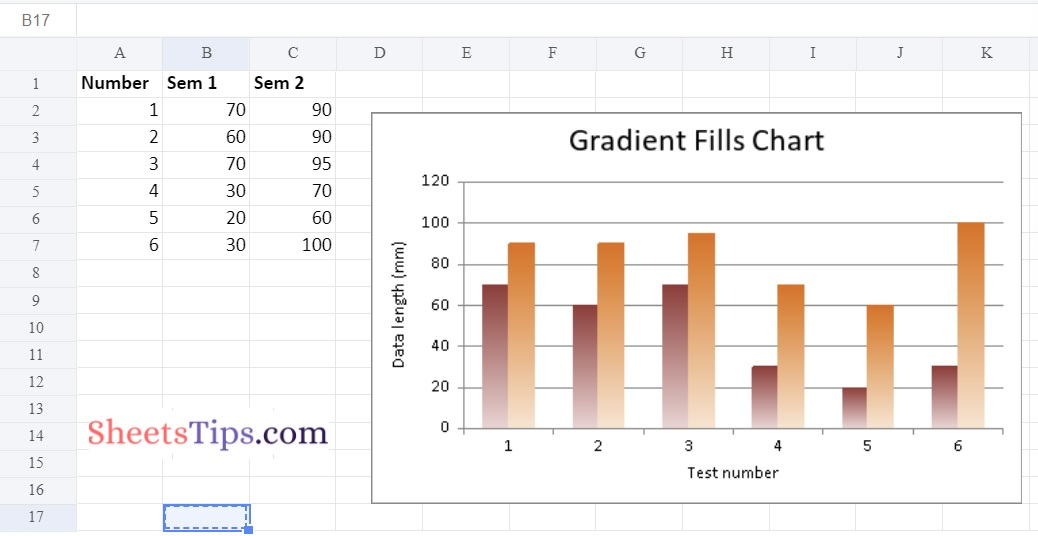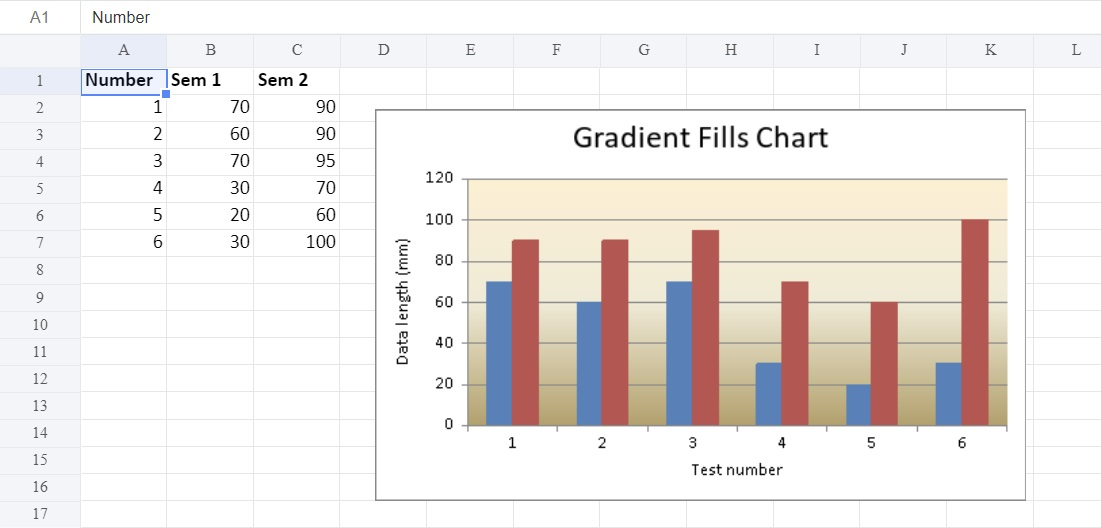# Python Program to Plot an Excel chart with Gradient fills using XlsxWriter module

XlsxWriter is a Python module that enables users to create, write, perform arithmetic operations on, and plot graphs on Excel files. Let’s look at how to use real-time data to plot a chart with gradient fills. Scroll down to learn more.

## Plot an Excel chart with Gradient fills using XlsxWriter module

Charts are made up of a series of one or more data points. The series are made up of references to cell ranges. To plot the charts on an excel sheet, first create a chart object of the desired chart type ( i.e Stock chart, etc.). After generating chart objects, insert data into them before adding them to the sheet object.

Install the xlsxwriter using the below code

`pip install xlsxwriter`

Looking in indexes: https://pypi.org/simple, https://us-python.pkg.dev/colab-wheels/public/simple/
Collecting xlsxwriter
|████████████████████████████████| 149 kB 5.0 MB/s
Installing collected packages: xlsxwriter
Successfully installed xlsxwriter-3.0.3

## Program to Plot an Excel chart with Gradient fills using XlsxWriter module in Python

### Method#1: Plotting Excel Chart with Gradient fills in columns

Approach:

• Import the xlsxwriter library using the import function.
• Pass the excel file path to the Workbook() function of xlsxwriter which is the file we want to create and store in a variable.
• Create the new Worksheet using the add_worsheet() function and apply it to the above workbook object and store it in a variable.
• Create a new Format object to format cells in spreadsheets using the add_format() method and apply it to the above workbook object.
• Here, we created a bold format object.
• Add the worksheet data that the charts will use.
• Write a row of heading data starting with ‘A1’ in the bold format using the write_row() function and apply it to the above worksheet object by passing the argument row number, headings list, and format.
• Write the column data that is starting from ‘A2’, ‘B2’, and ‘C2’ using the write_coulmn() function and pass data as an argument to it.
• Write a column of data starting from ‘A2’, ‘B2’, ‘C2’ respectively .
• Using the add_chart() method, create a chart object that can be added to a worksheet.
• Pass the type of chart as an argument to the add_chart() function and apply it to the above workbook object
Using the add series() method, you can add a data series with a gradient pattern to a chart.The gap is used to highlight the patterns.
• Set the title to the chartObject using the set_title() function by passing the title name as value and key as “name” (Here the arguments are object)
• Label the x axis using the set_x_axis() function by passing the Sem Number as value and key as “name” (Here the arguments are object)
• Label the y axis using the set_x_axis() function by passing the Data length as value and key as “name” (Here the arguments are object)
• set the legend to off using the set_legend() function
• Add a chartObject to the worksheet with the provided offset values in the top-left corner of a chartObject that is anchored to cell D2.
• Close the workbook Object using the close() function.
• The Exit of the Program.

Below is the Implementation:

```# Import the xlsxwriter library using the import function
import xlsxwriter

# Pass the excel file path to the Workbook() function of xlsxwriter
# which is the file we want to create and store it in a variable

# Create the new Worksheet using the add_worsheet() function and
# apply it to the above workbook object and store it in a variable

# Create a new Format object to format cells in spreadsheets using the add_format() method and apply it to the above workbook object.
# Here, we created a bold format object. .

# Add the worksheet data that the charts will use.
headingsData = ['Number', 'Sem 1', 'Sem 2']
worksheetData = [
[1, 2, 3, 4, 5, 6],
[70, 60, 70, 30, 20, 30],
[90, 90, 95, 70, 60, 100]
]

# Write a row of heading data starting with 'A1' in bold format using the write_row() function
# and apply on the above worksheet object
# by passing the argument rowNumber,headings list,and format.

# Write the column data that is starting from 'A2','B2','C2'
# using the write_coulmn() function
# and pass data as argument to it
# Write a column of data starting from
# 'A2', 'B2', 'C2' respectively .
newWorksheet.write_column('A2', worksheetData)
newWorksheet.write_column('B2', worksheetData)
newWorksheet.write_column('C2', worksheetData)

# Using the add_chart() method, create a chart object that can be added to a worksheet.
# Pass the type of chart as an argument to the add_chart() function and apply it to the above workbook object

# Using the add series() method, you can add a data series with a gradient pattern to a chart.
# The gap is used to highlight the patterns.

'name':	 '= Sheet1 !\$B\$1',
'categories': '= Sheet1 !\$A\$2:\$A\$7',
'values':	 '= Sheet1 !\$B\$2:\$B\$7',
'gradient': {'colors': ['# 963735', '# F1DCDB']}
})

'name':	 '= Sheet1 !\$C\$1',
'categories': '= Sheet1 !\$A\$2:\$A\$7',
'values':	 '= Sheet1 !\$C\$2:\$C\$7',
})

# Set the title to the chartObject using the set_title() function by passing the title name as value and key as "name" (Here the arguments are object)

# Label the x axis using the set_x_axis() function by passing the Sem Number as value and key as "name" (Here the arguments are object)
chartObject.set_x_axis({'name': 'Test number'})

# Label the y axis using the set_x_axis() function by passing the Data length as value and key as "name" (Here the arguments are object)
chartObject.set_y_axis({'name': 'Data length (mm)'})

# set the legend to off using the set_legend() function
chartObject.set_legend({'none': True})

# Add a chartObject to the worksheet with the provided offset values in the top-left corner of a chartObject that is anchored to cell D2.
newWorksheet.insert_chart('D2', chartObject,
{'x_offset': 25, 'y_offset': 10})

# Close the workbook Object using the close() function.
workbookObj.close()```

Output:### Method #2: Plotting  Excel Chart with Gradient fills in the plot area

To plot this type of chart on an excel sheet, use the set_plotarea() method using the chart object’s ‘gradient’ keyword parameter.

Below is the Implementation:

```# Import the xlsxwriter library using the import function
import xlsxwriter

# Pass the excel file path to the Workbook() function of xlsxwriter
# which is the file we want to create and store it in a variable

# Create the new Worksheet using the add_worsheet() function and
# apply it to the above workbook object and store it in a variable

# Create a new Format object to format cells in spreadsheets using the add_format() method and apply it to the above workbook object.
# Here, we created a bold format object. .

# Add the worksheet data that the charts will use.
headingsData = ['Number', 'Sem 1', 'Sem 2']
worksheetData = [
[1, 2, 3, 4, 5, 6],
[70, 60, 70, 30, 20, 30],
[90, 90, 95, 70, 60, 100]
]

# Write a row of heading data starting with 'A1' in bold format using the write_row() function
# and apply on the above worksheet object
# by passing the argument rowNumber,headings list,and format.

# Write the column data that is starting from 'A2','B2','C2'
# using the write_coulmn() function
# and pass data as argument to it
# Write a column of data starting from
# 'A2', 'B2', 'C2' respectively .
newWorksheet.write_column('A2', worksheetData)
newWorksheet.write_column('B2', worksheetData)
newWorksheet.write_column('C2', worksheetData)

# Using the add_chart() method, create a chart object that can be added to a worksheet.
# Pass the type of chart as an argument to the add_chart() function and apply it to the above workbook object

# Using the add series() method, you can add a data series with a gradient pattern to a chart.
# The gap is used to highlight the patterns.

'name':	 '= Sheet1 !\$B\$1',
'categories': '= Sheet1 !\$A\$2:\$A\$7',
'values':	 '= Sheet1 !\$B\$2:\$B\$7'
})

'name':	 '= Sheet1 !\$C\$1',
'categories': '= Sheet1 !\$A\$2:\$A\$7',
'values':	 '= Sheet1 !\$C\$2:\$C\$7'
})
# Set the Gradient for the plot area using the set_plotarea() function
chartObject.set_plotarea({
'gradient': {'colors': ['# FFEFD1', '# F0EBD5', '# B69F66']}
})

# Set the title to the chartObject using the set_title() function by passing the title name as value and key as "name" (Here the arguments are object)

# Label the x axis using the set_x_axis() function by passing the Sem Number as value and key as "name" (Here the arguments are object)
chartObject.set_x_axis({'name': 'Test number'})

# Label the y axis using the set_x_axis() function by passing the Data length as value and key as "name" (Here the arguments are object)
chartObject.set_y_axis({'name': 'Data length (mm)'})

# set the legend to off using the set_legend() function
chartObject.set_legend({'none': True})

# Add a chartObject to the worksheet with the provided offset values in the top-left corner of a chartObject that is anchored to cell D2.
newWorksheet.insert_chart('D2', chartObject,
{'x_offset': 25, 'y_offset': 10})

# Close the workbook Object using the close() function.
workbookObj.close()```

Output:Python is one of the powerful programming languages that help us automate excel. It has various modules with the help of which we can easily create charts and many other things without launching the Excel files. Stay tuned to SheetsTips.Com to learn more about how to automate Excel with Python.